• ×
الأربعاء 29 نوفمبر 2023 | 12-19-2022
×

# المجال الكهربائي Electric FieldIn this lecture we will learn about electric field, the properties of the electric field lines, and the superposition principle. You can watch the following video or read the written tutorial below.

*

Two electrically charged objects exert a force>What is an Electric Field?

If we have a charged object we can actually predict what will happen to other*electric charges*when they are brought close to that object.

A charged object modifies the space around it, forming an electric field. The electric field has an ability to act with a force>When we talked about*Coulomb’s Law*we observed the force between two charges as an action between two objects, and now we’ll observe the force as an action that the electric field exerts>Michael Faraday was a British scientists, and was the first person to introduce the concept of electric field. A field can be:

• Any quantity that can be given a value at all points in space around the source of the field; or
• A “region of influence”.

## How Electric Fields Work?

First of all, the strength of the electric field is a vector quantity, having a magnitude and a direction. The direction of the* field at a point is actually the same direction as the force acting>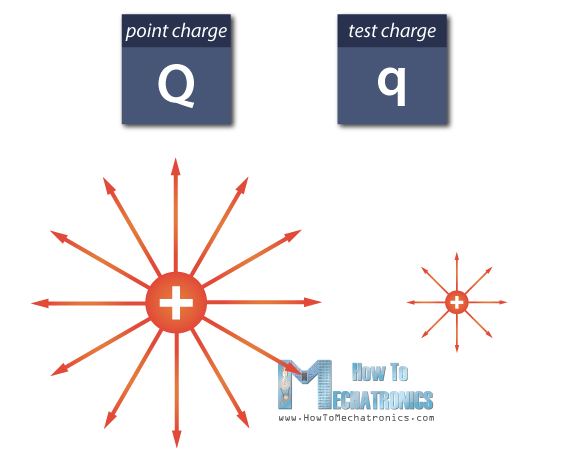Let’s say there is a positive point charge, denoted by capital Q. In order to see the effect this charge has>The reason we bring in a small test charge is because we want a value that is independent of the size of the test charge, and depends>Test charge is always positive by definition. Both charges create electric fields, but big Q has a much larger magnitude than small q. The point charge Q modifies the space around it and sets up an electric field.

## Electric Field Formula

Now we can measure the field.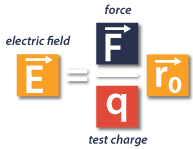The electric field generated by a charged object, is in fact the electric force between the object and the test charge, divided by the magnitude of that test charge. Coulomb’s Law will help us find the force.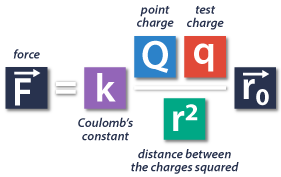So, in place of capital F, we’ll use the Coulomb’s Law equation.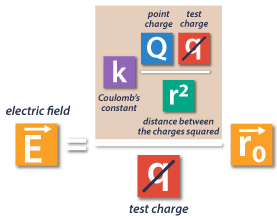Here, we can notice that small q appears twice in this equation, so it cancels out, and we get an equation that includes>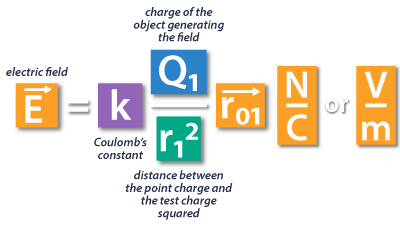Finally, the strength of an electric field created by a point charge is equal to the Coulomb’s constant multiplied by the charge>Here we have positive point charge and positive test charge, and the result has a positive sign, meaning that the positively charged object will repel the positive test charge. That’s why the electric field diagram has arrows pointing outward.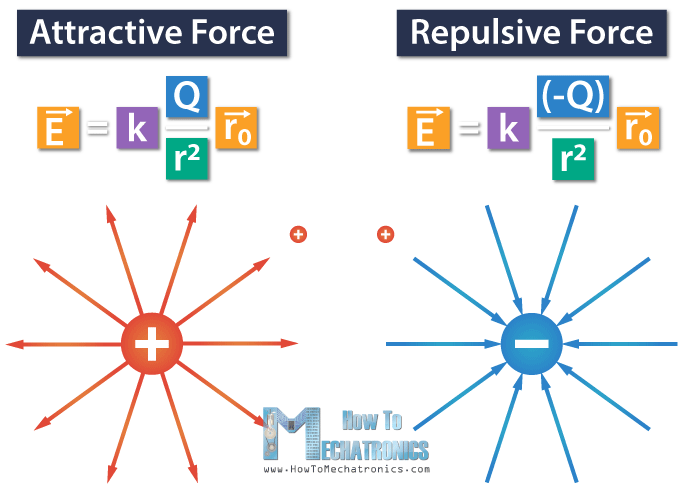For negative point charge, and positive test charge we have an attractive force, which is shown by the negative sign in the equation.

The electric field line diagram for negatively charged object would look like that of a positively charged object, but with the arrows pointing inward, which means that*the negatively charged object Q would attract the positive test charges.

The unit of measurement for electric field is Newtons/Coulomb, or Volts/Meter.

Electric field exists even if there is no test charge nearby to measure it. Similarly, a gravitational field surrounds the earth, even if there is no “test mass” nearby to measure it.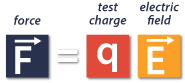If we know the value of the point charge and the value of the strength of the electric field, we can easily calculate the force, simply by multiplying those values.

## Example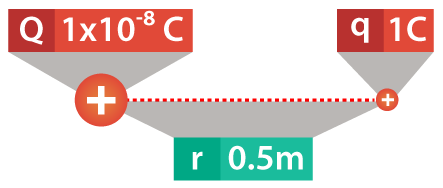For example, we have a point charge Q equal to 1×10-8 Coulombs. Also, we have a test charge of 1 Coulomb at a 50 cm distance. So, what is the electric field 50 cm away from the point charge?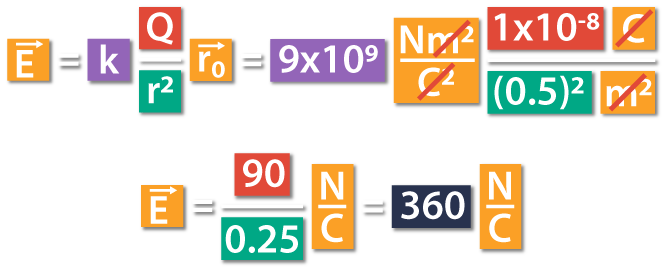The electric field at that point is going to be Coulomb’s constant multiplied by the charge generating the field, divided by the distance between the point charge and the test charge squared. This is equal to 9×109 Nm2/C2 times 1×10-8 C divided by (0.5m)2.

Here we can cancel out meters and Coulombs, which gives us Newtons/Coulomb. This is equal to 90 divided by 0.25 Newtons/Coulomb. Finally, the magnitude of the electric field that the point charge generated at 50 cm distance is 360 Newtons/Coulomb.The force exerted>Here we can notice that both the point charge and the test charge are positive, so the force will be repulsive, meaning that the direction of the force will be outwards.

## Superposition Principle

Superposition principle applies to electric fields as well.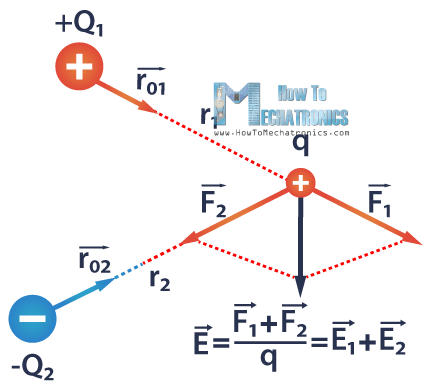Let’s say we have two point charges Q1 and Q2, and we bring a positive test charge q nearby. Q1 is positive, and Q2 is negative charge. The force that Q1 applies>The superposition principle can help us calculate the total electric field created by multiple point charges, which is simply a sum of all the individual fields.

In other words, the total electric field at a point from a system of charges acting>Using the equation, we can calculate the total electric field E, which is equal to E1 E2: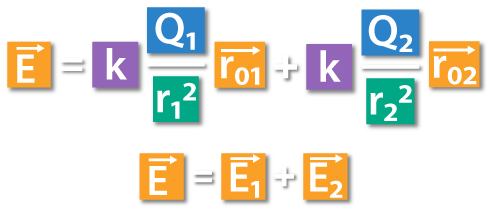## Electric Field Lines

A small positive test charge q placed at various points near Q would be repelled (or attracted) by Q and would move along the lines. These vectors are called lines of force, or electric field lines, a concept first introduced by Michael Faraday.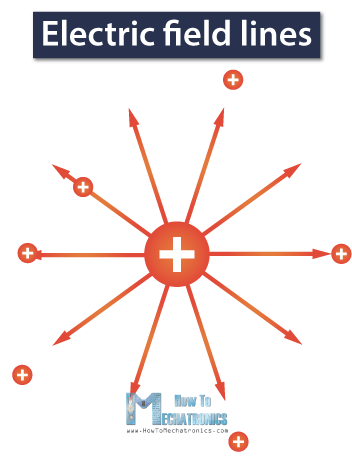They show the direction and the magnitude of the force applied>Properties of Electric Field Lines

Let’s take a look at the field between two charges of similar magnitude but opposite sign. They form an electric dipole.

Now let’s connect their electric fields and create a total electric field.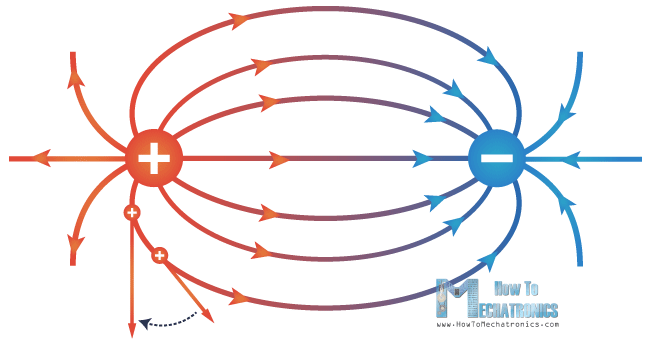If we pay attention to this total electric field it will reveal four important properties of electric field lines.

1. The lines always begin>The field lines must be tangent to the direction of the field at any point.
2. The closeness of the lines indicates the strength of the field. The greater the line density, the greater the magnitude of the field, and opposite.
3. The lines never cross. A charge cannot go in two directions at the same time.

OK, that’s all for this tutorial. I hope you enjoyed it and learned something new. In the next Basic Electronics tutorial we will talk about Work and Potential.

المصدر*

محدث
07-16-2019

## روابط التنزيل

من فضلك قم بكتابة تعليق لتظهر لك روابط التحميل

بواسطة :
06-18-2019 12:25 صباحاً  2  0  3.1K  439
التعليقات ( 2 )
الترتيب بـ
الأحدث
الأقدم
الملائم
•TLILI 05-20-2021 03:36 صباحاً

•Mahmoud 02-23-2020 10:05 مساءً

Good file.

0 | 3.3K | 0

1 | 2.0K | 2

0 | 2.6K | 258

0 | 2.5K | 1

0 | 2.4K | 1

4 | 2.4K | 2056